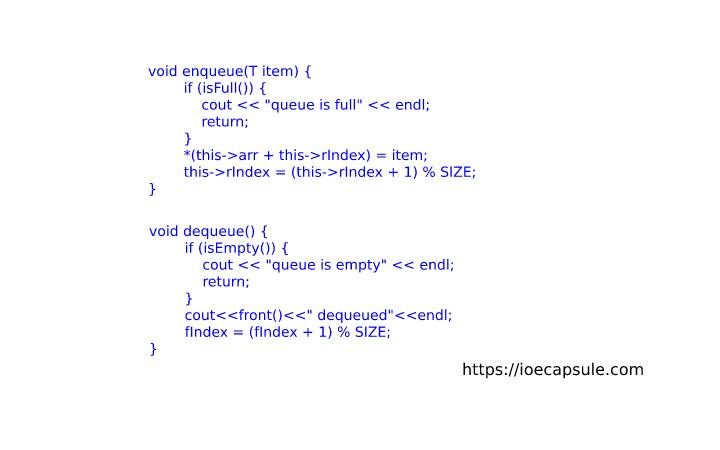### Queue: Template Implementation Of Circular Queue Using C++Data Structures

A circular queue is the circular array implementation in which we view array as circle rather than linear . In this implementation, the first element of array is immediately followed by its last element. By increment either rear or front index, we can always insert an item unless the array is fully occupied.

In previous article, we discussed about algorithms of insertion and deletion of circular queue. If you missed, feel free to check that out.

Queue: Circular Queue Implementation

Here, we are going to write some c++ code implementing circular queue. The code is pretty simpler one so if you like to modify it, feel free to copy and modify as you like.

#### Template Implementation Circular Queue Using C++

``````
#include<iostream>
#define SIZE 5

using namespace std;

template < typename T > class Queue {
private:
T * arr;
int fIndex, rIndex;
public:
Queue() {
arr = new T[SIZE];
fIndex = 0;
rIndex = 0;
}

bool isFull() {
return (rIndex + 1) % SIZE == fIndex;
}

bool isEmpty() {
return rIndex == fIndex;
}

void enqueue(T item) {
if (isFull()) {
cout << "queue is full" << endl;
return;
}
*(this->arr + this->rIndex) = item;
this->rIndex = (this->rIndex + 1) % SIZE;
}

void dequeue() {
if (isEmpty()) {
cout << "queue is empty" << endl;
return;
}
cout<<front()<<" dequeued"<<endl;
fIndex = (fIndex + 1) % SIZE;
}

T front(){
return isEmpty() ? NULL : *(arr + fIndex);
}
};

int main() {
bool quit = false;
Queue < int > queue;
int temp;
do {
cout << "====================================" << endl;
cout << "select option :" << endl;
cout << "1 for enqueue" << endl;
cout << "2 for dequeue" << endl;
cout << "3 for front item" << endl;
cout << "4 for exit" << endl;
int ch;
cin >> ch;
cout << "====================================" << endl;
switch (ch) {
case 1:
cout << "enter item to enqueue:" << endl;
cin >> temp;
queue.enqueue(temp);
break;
case 2:
queue.dequeue();
break;
case 3:
if (queue.isEmpty()) {
cout << "queue is empty" << endl;
} else {
cout << "front: " << queue.front() << endl;
}
break;
case 4:
quit = true;
break;
default:
cout << "invalid selection" << endl;
break;
}
} while (!quit);
return 0;
}
``````